# Best fit sigmoid curve

## How to Find the Best Fit Sigmoid Curve

Now the question is which is the actual sigmoid curve and how to find the best fit sigmoid curve for the given data points.

As shown in the below diagram, there can be multiple sigmoid curves that can be defined for a set of data points. You need to find an optimal sigmoid curve so that the corresponding fit can be a best-fit curve.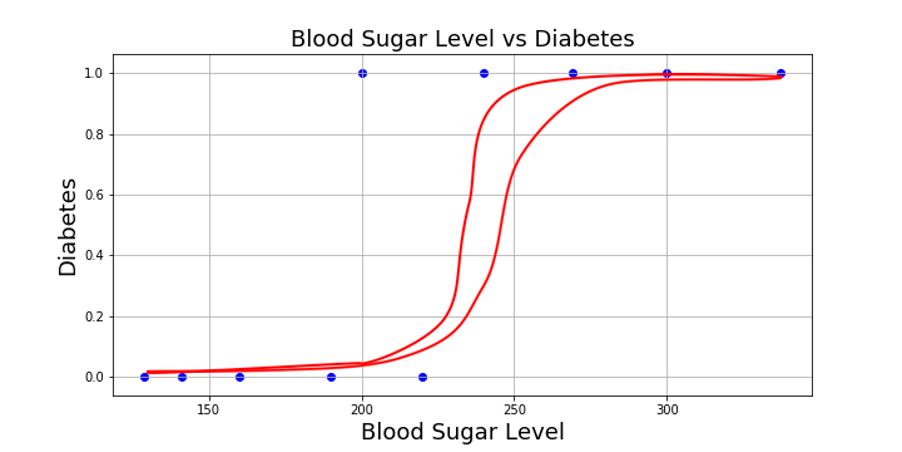In the case of Linear regression, we used the derivative method to find optimal β0 and β1 from cost function (RSS). (Follow this link for more)

Let’s derive our cost function for the sigmoid curve.

In this case, we would like to keep the value of data points that are non-diabetic (p1, p2, p3, p4, and p6) to be as low as possible. Whereas the values for diabetic data points to be as high as possible. Here higher value means higher probability.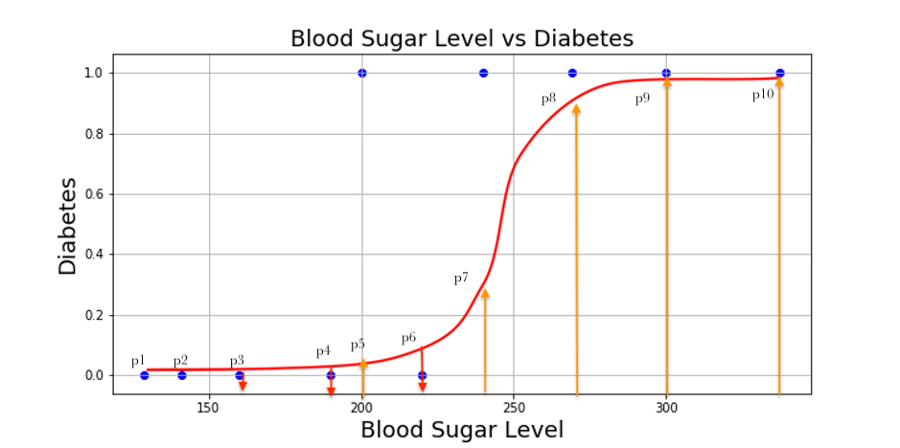Alternatively, Instead of minimizing p1, we can say to maximize (1-p1). In this way, we can create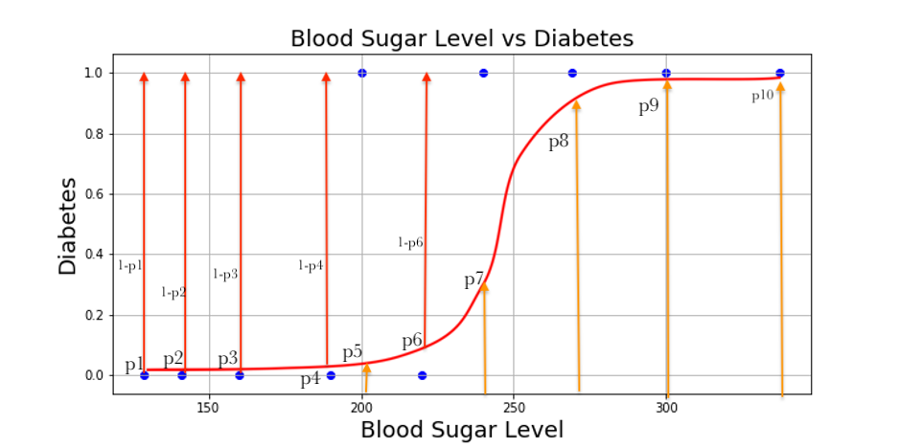The best-fitting sigmoid curve defined by the curve whose products are maximum.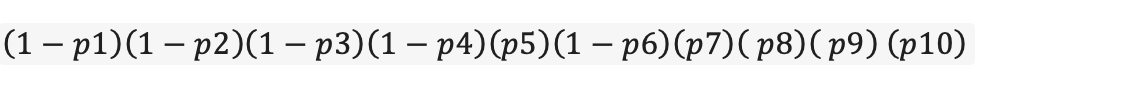This function also called the Likelihood function. Let’s write the likelihood function mathematically.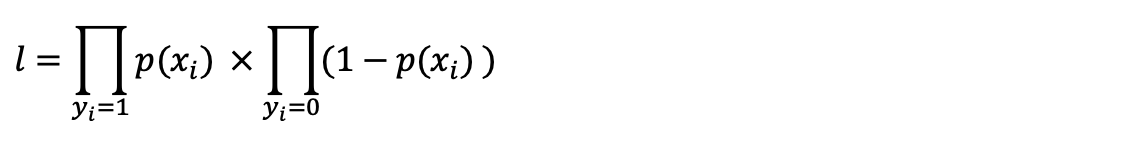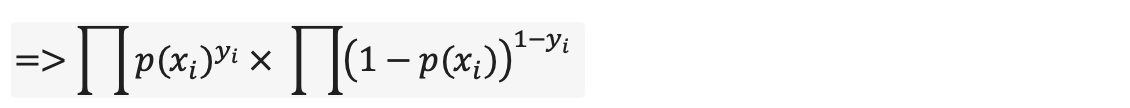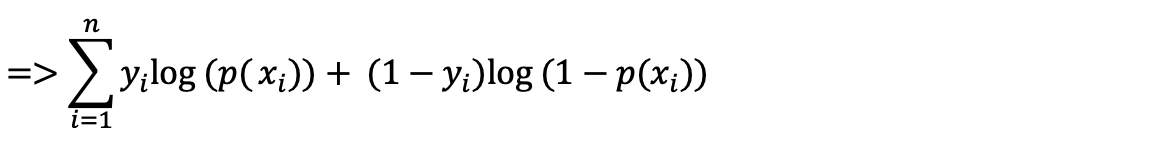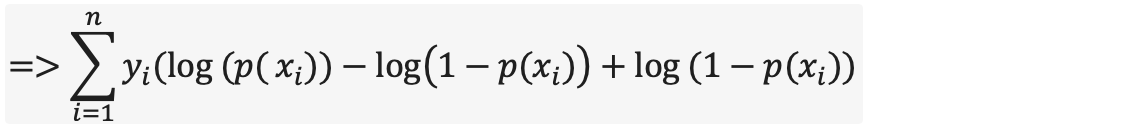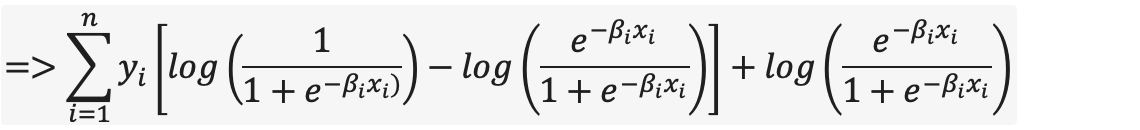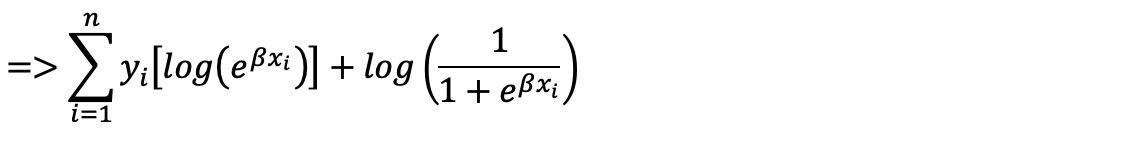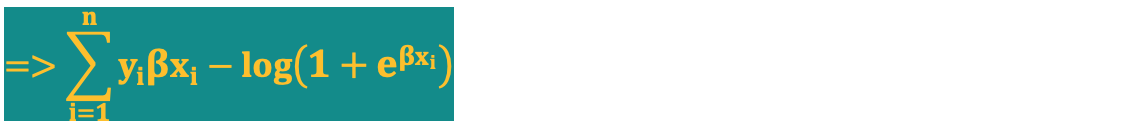This is the function which we will maximize to get the optimal value of β.

Conclusion:

In this blog, you learned about, how to find the best fit sigmoid curve and the basic math around the same. Please let us know your feedback below in the comment section.